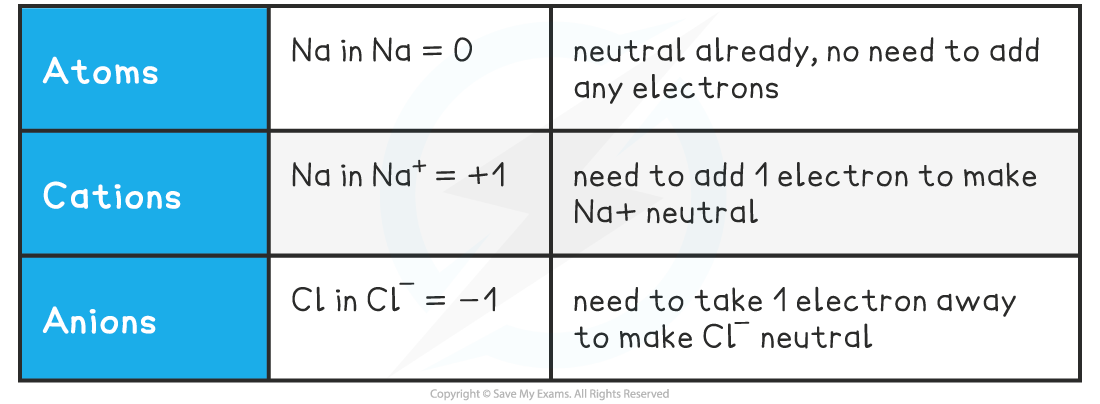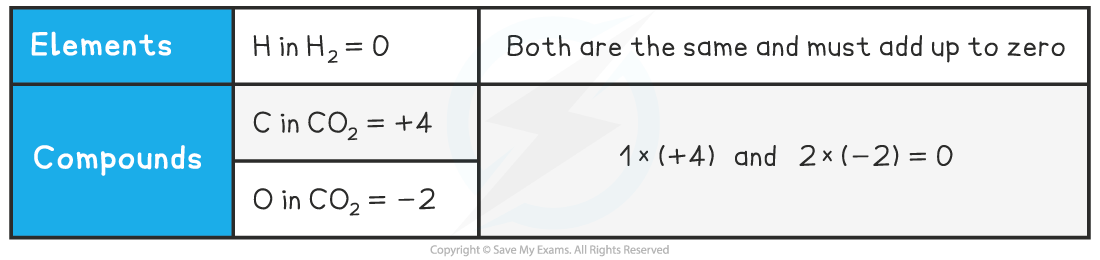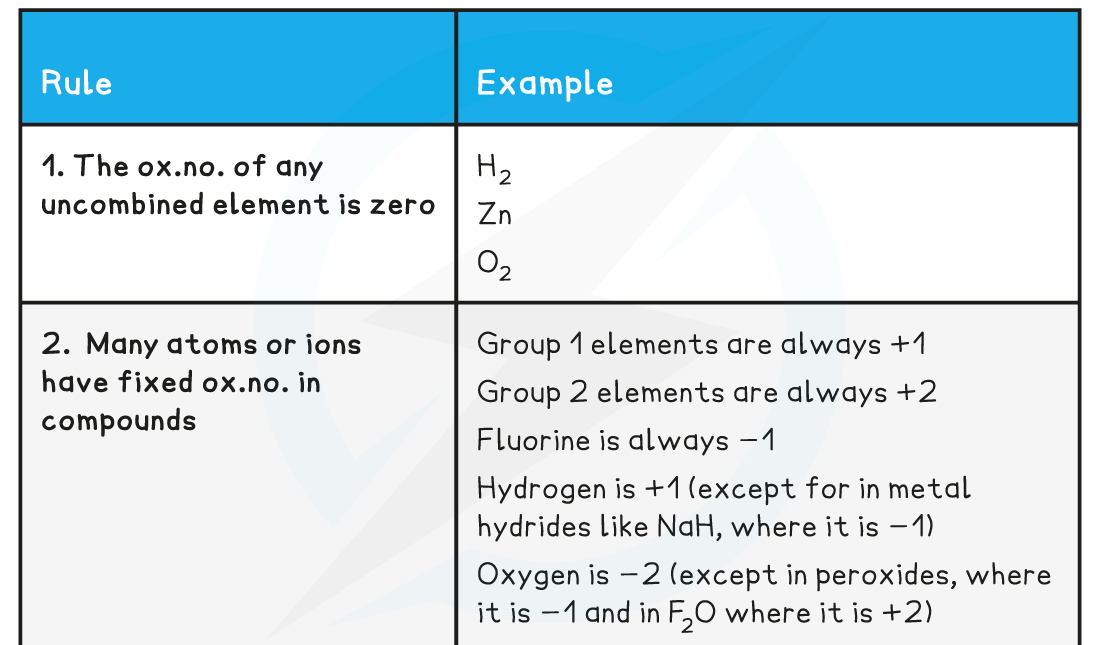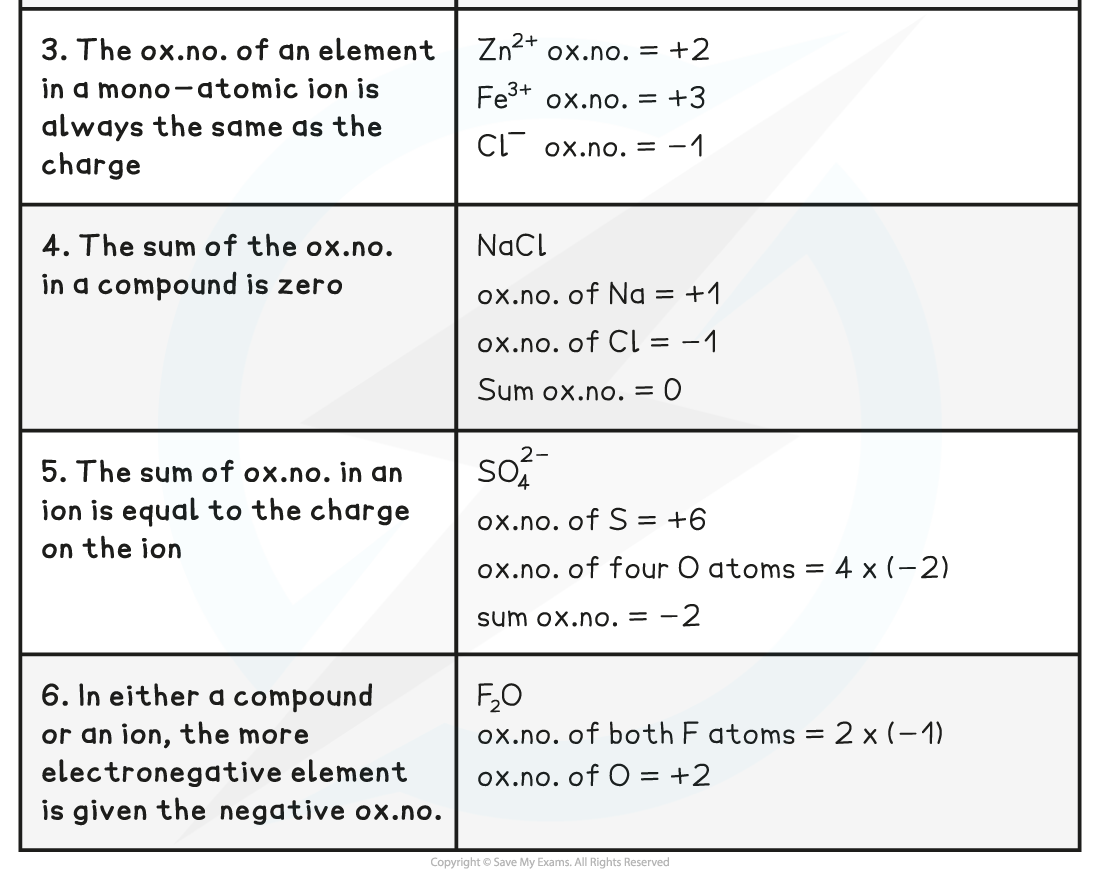# IB DP Chemistry: SL复习笔记9.1.1 Oxidation & reduction

### Oxidation & Reduction

• There are three definitions of oxidation and reduction  used in different branches of chemistry
• Oxidation and reduction can be used to describe any of the following processes

Definitions and Examples of Oxidation & Reduction Table#### Oxidation Numbers

• The oxidation number of an atom is the charge that would exist on an individual atom if the bonding were completely ionic
• It is like the electronic ‘status’ of an element
• Oxidation numbers are used to...
• tell if oxidation or reduction has taken place
• work out what has been oxidised and/or reduced
• construct half equations and balance redox equations

#### Atoms and simple ions

• The oxidation number is the number of electrons which must be added or removed to become neutral
• The oxidation number is always written with the charge before the number

Oxidation Number of Simple Ions Table#### Worked Example

What are the oxidation states of the elements in the following species?

a) C                b)  Fe3+                       c)  Fe2+             d) O2-             e)  He                          f)  Al3+

a) 0     b) +3    c) +2    d) -2    e) 0    f) +3

• So, in simple ions, the oxidation number of the atom is the charge on the ion:
• Na+, K+, H+ all have an oxidation number of +1
• Mg2+, Ca2+, Pb2+ all have an oxidation number of +2
• Cl-, Br-, I- all have an oxidation number of -1
• O2-, S2- all have an oxidation number of -2

#### Molecules or Compounds

• In molecules or compounds, the sum of the oxidation numbers on the atoms is zero

Oxidation Number in Molecules or Compounds Table• Because CO2 is a neutral molecule, the sum of the oxidation states must be zero
• For this, one element must have a positive oxidation number and the other must be negative

How do you determine which is the positive one?

• the more electronegative species will have the negative value
• electronegativity increases across a period and decreases down a group
• O is further to the right than C in the periodic table so it has the negative value

How do you determine the value of an element's oxidation state?

• from its position in the periodic table and/or
• the other element(s) present in the formula

### Variable Oxidation Numbers

• Many atoms, such as S, N and Cl, can exist in a variety of oxidation states
• The oxidation number of these atoms can be calculated by assuming that the oxidation number of the other atom is fixed
• Here are six rules to deduce the oxidation number of an element

Oxidation Number Rules Table#### Exam Tip

Oxidation number and oxidation state are often used interchangeably, though IUPAC does not distinguish between the two terms.Oxidation numbers are represented by Roman numerals according to IUPAC